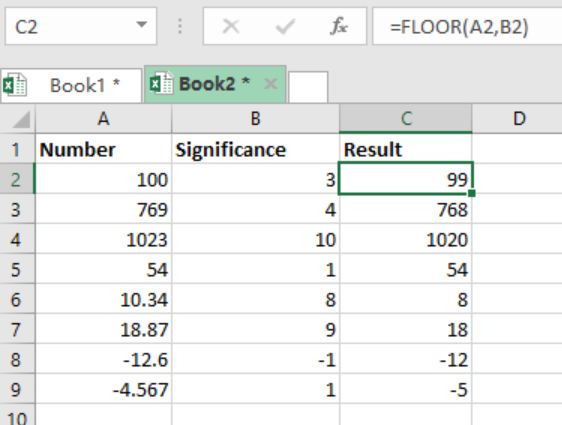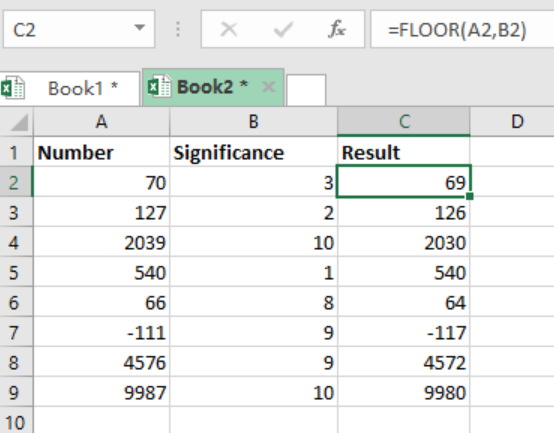Get instant live expert help with Excel or Google Sheets“My Excelchat expert helped me in less than 20 minutes, saving me what would have been 5 hours of work!”

#### Post your problem and you’ll get expert help in seconds.

Your message must be at least 40 characters
Our professional experts are available now. Your privacy is guaranteed.

# Excel FLOOR Function

We can use Excel FLOOR function to round numbers down to the nearest specified multiple. To learn how to use this function, read this post as it provides a step-by-step guide on how to round down numbers using the FLOOR function.Figure 1: Use of Excel FLOOR function

## Syntax of the formula

`=FLOOR(number, multiple)`

Where;

• Number- refers to the number to be rounded
• Multiple- multiple to be used when rounding

## Explanation of the formula

When we want to round a number down to a given multiple, we use the Excel FLOOR function. It is important to note that in excel, we can round numbers either up or down. When rounded up, the numbers will get bigger after rounding. But when rounded down, the numbers will get smaller after rounding. Excel FLOOR function is best described as rounding a number either away or towards zero.

## ExampleFigure 2: Rounding down numbers with Excel FLOOR function

The FLOOR function works in the same way the MROUND function does, with the only difference being that it always round numbers down to the desired number. For positive numbers, they will be rounded towards zero, while negative numbers will be rounded away from zero. This function is most useful in setting up rates, discounts and more.

## Instant Connection to an Expert through our Excelchat Service

Most of the time, the problem you will need to solve will be more complex than a simple application of a formula or function. If you want to save hours of research and frustration, try our live Excelchat service! Our Excel Experts are available 24/7 to answer any Excel question you may have. We guarantee a connection within 30 seconds and a customized solution within 20 minutes.

### Did this post not answer your question? Get a solution from connecting with the expert.Another blog reader asked this question today on Excelchat:## Subscribe to Excelchat.coAnother blog reader asked this question today on Excelchat: